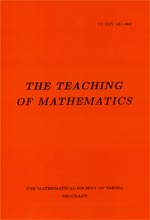﻿ The Teaching of Mathematics - Instructions for Authors ﻿
 THE TEACHING OF MATHEMATICSLagrange's formula for vector-valued functions Milosav M. Marjanović and Zoran Kadelburg AbstractIn this paper we derive a variant of the Lagrange's formula for the vector-valued functions of severable variables, which has the form of equality. Then, we apply this formula to some subtle places in the proof of the inverse function theorem. Namely, for a continuously differentiable function $f$, when $f'(a)$ is invertible, the points $a$ and $b = f(a)$ have open neighborhoods in the form of balls of fixed radii such that $f$, when restricted to these neighborhoods, is a bijection whose inverse is also continuously differentiable. To know the radii of these balls seems to be something hidden and tricky, but in the proof that we suggest the existence of such neighborhoods is ensured by the continuity of the involved correspondences.Keywords: Mean value theorem; inverse mapping theorem. Pages:  81$-$88 Volume  XX ,  Issue  2 ,  2017

﻿